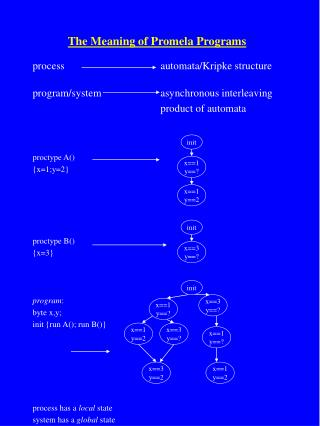DownloadDownload PresentationThe Meaning of Promela Programs

# The Meaning of Promela Programs

Download Presentation## The Meaning of Promela Programs

- - - - - - - - - - - - - - - - - - - - - - - - - - - E N D - - - - - - - - - - - - - - - - - - - - - - - - - - -
##### Presentation Transcript

1. The Meaning of Promela Programs init x==1 y==? x==1 y==2 init processautomata/Kripke structure program/systemasynchronous interleaving product of automata proctype A() {x=1;y=2} proctype B() {x=3} program: byte x,y; init {run A(); run B()} process has a local state system has a global state x==3 y==? init x==3 y==? x==1 y==? x==1 y==2 x==3 y==? x==1 y==? x==3 y==2 x==1 y==2

2. Count==0 Count==1 proctype A() {bit count =0; do :: (count == 0) -> count=count+1 :: (count!=0) -> count=count-1 od } init { run A()}

3. []  [0,0] chan ch =  of bit; proctype A() {bit x=0; do :: ch!x :: ch?x od } init {run A()} OR proctype A() {do :: ch!x od} proctype B() {do :: ch?x od} init {atomic{ run A(); run B()}}

4. Message Passing Channels • allow transfer of data from one process to another chan one =  of int chan two =  of {bool, int, int} • writing a message • one!154 • queue!x • two!false,19393,2 only executable when the channel is not full • reading a message • one?var1 • two?on,num1,num2 only executable when the channel is not empty

5. Message Passing • prefefined operations len(channel) - returns no. of messages len of empty channel blocks full - returns status (i.e. is channel full) empty - returns status (i.e. is channel empty) Also, nfull and nempty.

6. Message Passing • channels can also be passed as messages! • Proctype A(chan q1) • { chan q2; • q1?q2; • q2!123 • } • Proctype B(chan q3) • {int x; • q3?x; • printf(“x = %d\n”, x) • } • init • {chan qname =  of {chan}; • chan qforb =  of {int}; • run A(qname); run B(qforb); • qname!qforb • }

7. Message Passing • non-destructive read q1?[message]; message == somethinggood -> something else q1?[m] returns 1if q1?m is executable, 0 otherwise. No side-effects, receive is evaluated, not executed. • race conditions - BE CAREFUL! (len (channelA) < Max) -> channelA!message concurrently with channelA! Message (len (channelA) > 0) -> channelA?x concurrently with channelA? x